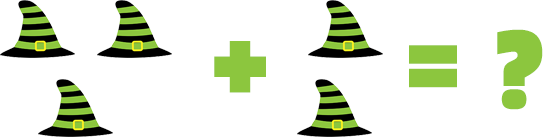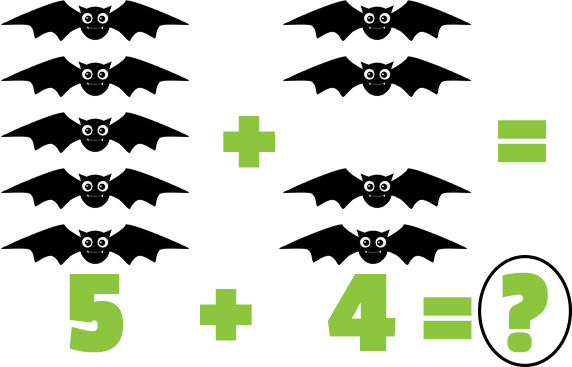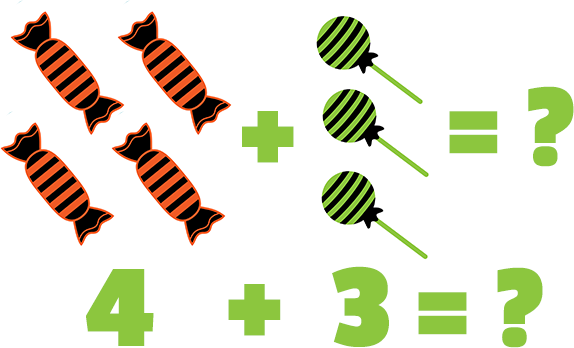Description: Add numbers up to 10.

Tags: Kindergarten, Halloween, Addition, CCSS.MATH.CONTENT.K.OA.A.1, Kindergarten Math Problems

Question 1 (Multiple Choice, 10 points)

What is 3 + 2?Question 2 (Multiple Choice, 10 points)

5+4=?Question 3 (Multiple Choice, 10 points)

What is 2 + 1?

Question 4 (Matching, 10 points)

What is 3 + 3?

 3+3= --select-- 5 7 8 6
Question 5 (Multiple Choice, 10 points)

4+3=?